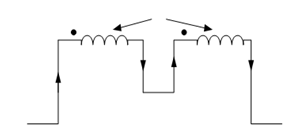## Series Opposing Assignment Help

Assignment Help: >> Inductive Coupling in Series - Series Opposing

Series Opposing:

Figure illustrates two coils coupled in series opposition as their fluxes are in opposite direction as per dot convention.Figure: Inductive Coupling in Series (Flux Opposing)

Let us consider coil 1 and coil 2 individually.

Coil 1

Self-induced e.m.f e  =- L1  (di /dt)

Mutually induced e.m.f e1′ = M (di /dt)  [due to change of current in coil 2]

Coil 2

Self-induced e.m.f e2  =- L2  (di /dt)

Mutually induced e.m.f e2′ = M(di/dt) [due to change of current in coil 1]

Therefore the total induced e.m.f of the combination can be written as

e = e1  + e2  + e1′ + e2

e =- L1  (di /dt) - L2( di/ dt) + 2 M (di/dt) =- (L1 + L2  - 2M ) (di/dt)

If L is the equivalent inductance of the combination, then we may write

e =- L( di/ dt)

From Eqs (39) and (40), we get

- L (di / dt )= - (L1  + L 2 - 2M ) (di /dt)

or, L = L1  + L2  - 2M#### Assured A++ Grade

Get guaranteed satisfaction & time on delivery in every assignment order you paid with us! We ensure premium quality solution document along with free turntin report!

All rights reserved! Copyrights ©2019-2020 ExpertsMind IT Educational Pvt Ltd